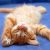# Matlab使用interp2裁剪倾斜（旋转）矩形块

在图像处理中，我们常常需要裁剪出图像中的一个矩形块，matlab提供的imcrop函数可以裁剪出矩形块，但是如果我们想裁剪倾斜的矩形块，该函数将无能为力。于是博主贡献了自己的代码。

核心是使用interp2进行插值，没什么好介绍的，代码title使用说明也写的比较清楚，直接贴代码好了。

function [ out ] = get_pixels( im, pos, sz, theta )
%UNTITLED 此处显示有关此函数的摘要
%   im:    输入的uint8类型图像
%   theta: 所求patch相对于原图，顺时针旋转的弧度数
%   pos:   要截取图像块在原图中的中心坐标
%   sz:    要截取图像块的大小
if isscalar(sz)
sz = [sz, sz];
end

c = sz/2;
im = single(im);
[X, Y] = meshgrid(1:sz(2), 1:sz(1));
X = X - c(2);
Y = Y - c(1);
ct = cos(theta);
st = sin(theta);
Xi = pos(2) + ct*X - st*Y;
Yi = pos(1) + st*X + ct*Y;

if size(im,3)==3
out(:,:,1) = interp2(im(:,:,1), Xi, Yi, 'linear');
out(:,:,2) = interp2(im(:,:,2), Xi, Yi, 'linear');
out(:,:,3) = interp2(im(:,:,3), Xi, Yi, 'linear');
else
out = interp2(im(:,:,1), Xi, Yi, 'linear');
end

out = uint8(out);


输入如果不是uint8图像，自行修改，不过注意interp2只能输入single或者double。

OK，See You Next Chapter！

### 《Matlab使用interp2裁剪倾斜（旋转）矩形块》有2条评论

1.请问可以举一个完整的例子吗？参数pos的输入形式应该是怎样的呢？

回复
•% im: 输入的uint8类型图像

% theta: 所求patch相对于原图，顺时针旋转的弧度数

% pos: 要截取图像块在原图中的中心坐标 = [center_x, center_y]

% sz: 要截取图像块的大小 = [height, width]

回复